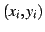## 2 Automatic generation of gait control tables

Control tables are generated using a wave propagation model. The algorithm is as follows (figure). Having a waveform in its initial state,(in the figure, sinusoidal waves are drawn, but other waveforms could be used) and a worm with all its articulations over theaxis (figure-1). Letbe the coordinates of the articulation, at some instant. The angular position vector for the initial time,, is calculated fitting the articulations to the wave, so thatfor all i. The distance L between articulations is maintained. It could be said that the worm fits the wave'' (figure-2). Next, the wave is shifted (instant. Figure-3) and the worm fits the wave again, obtaining(figure-4). Points 3 and 4 are repeated until the wave reach its initial phase. Afterinstant of time, all the vector that comprises the table are generated.By means of this algorithm, control tables are obtained, regardless of the waveform used,. In the locomotion test, sinusoidal and semi-sinusoidal waves (just the positive part of the sinusoidal wave) have been used.

Juan Gonzalez 2004-10-08